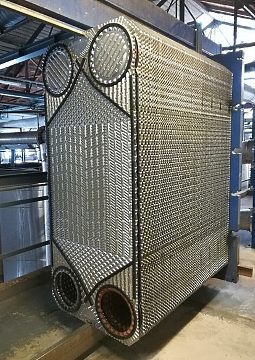calculator.org

# property>heat transfer coefficient

## What is the Heat Transfer Coefficient?In chemical and mechanical engineering, the heat transfer coefficient is used for calculating heat transfer between a fluid and a solid, between fluids separated by a solid, or between two solids, and is the inverse of thermal insulance. The heat transfer coefficient has the SI units W/(m2K) and is calculated in the following way:

h = ∆Q/(A * ∆T * ∆t)

where h is the heat transfer coefficient, ∆Q is the heat input into the system or the heat lost, A is the surface area where the heat is transferred, ∆T is the temperature difference between the sold surface and the surrounding fluid area, and ∆t is change in time comprising the time period in which the heat transfer has occurred.

Depending on the way in which heat is transferred, the heat transfer coefficient is calculated in a variety of ways. Most solid substances have a known thermal conductivity which can be used as a basis for calculating the heat transfer coefficient. A very common engineering problem is the heat transfer between a fluid and a solid surface.The most common way of doing this is by dividing the thermal conductivity of the convection fluid by a length scale. It is also common to calculate the coefficient with the Nusselt number (one of a number of dimensionless groups used in fluid dynamics).

Under forced convection circumstances (a type of heat transfer in which the movement of fluid is generated by an external source rather than just the buoyancy of the heated fluid), one can determine the heat transfer coefficient by using the Dittus-Boelter correlation. This can be helpful for the purpose of designing heat exchangers, which are devices that are designed to transfer heat from one medium to another for commercial purposes. One example of a heat exchanger is the radiator in your car, but there are many others. Heat exchangers are used in refrigeration, air conditioning, chemical process plant, and space heating, to name a few. Although the Dittus-Boelter correlation is not perfectly accurate, it is useful for some applications and is estimated to be, on accurate to within 15 percent. Using the Dittus-Boelter correlation the heat transfer coefficient can be calculated in the following way using two further dimensionless groups, the Reynolds number and the Prandtl number:

h = (kw/DH)*Nu

where kw is the thermal conductivity of the liquid, DH is the Hydraulic diameter, and Nu is the Nusselt Number which is determined by the following equation:

Nu = 0.023*Re0.8*Prn

In this equation, Re is the Reynolds number which equates to:

Re = (m* DH)/(μ*A)

And Pr is the Prandtl number, equating to:

Pr = (Cp* μ)/kw

For the Reynolds number, m is equal to the mass flow rate, and A is a cross sectional area of flow taken from the tube. For the Prandtl number, Cp is equal to heat capacity (assuming a constant pressure), and in both equations, μ is the viscosity of the fluid in consideration. The Reynolds number is a measure of the relative importance of viscous and inertial forces (which will bring about turbulence). Once we have all of these factors, we can get a decent estimation of the heat transfer rate through a particular kind of heat exchanger we plan on designing.

The equation for the heat transfer rate Q, is written as follows:

Q = 1/((1/h) + (t/k))AΔT

where t is the thickness of the wall through which the heat is transferred, A is the area of transfer, and k is the thermal conductivity of the medium.

Heat exchangers are similar to electrical circuits in a few senses. Heat flow is additive along parallel "circuits" and inversely additive along heat transfer processes connected in series. The heat transfer coefficient also works in this way. For heat transfer processes connected in parallel, the total value of h is equal to:

h = h1 + h2 + h3 + ... + hn

where each subprocess has its own heat transfer coefficient. For heat transfer processes connected in series, the equation is written as:

h = 1/h1 + 1/h2 + 1/h3 + ... + 1/hn

Bookmark this page in your browser using Ctrl and d or using one of these services: (opens in new window)